# Cost Volume Profit Analysis

Cost-volume-profit (CVP) analysis is used to determine how changes in costs and volume affect a company’s operating income and net income. In performing this analysis, there are several assumptions made, including:

• Sales price per unit is constant.
• Variable costs per unit are constant.
• Total fixed costs are constant.
• Everything produced is sold.
• Costs are only affected because activity changes.
• If a company sells more than one product, they are sold in the same mix.

CVP analysis requires that all the company’s costs, including manufacturing, selling, and administrative costs, be identified as variable or fixed.

### Contribution margin and contribution margin ratio

Key calculations when using CVP analysis are the contribution margin and the contribution margin ratio. The contribution margin represents the amount of income or profit the company made before deducting its fixed costs. Said another way, it is the amount of sales dollars available to cover (or contribute to) fixed costs. When calculated as a ratio, it is the percent of sales dollars available to cover fixed costs. Once fixed costs are covered, the next dollar of sales results in the company having income.

The contribution margin is sales revenue minus all variable costs. It may be calculated using dollars or on a per unit basis. If The Three M’s, Inc., has sales of \$750,000 and total variable costs of \$450,000, its contribution margin is \$300,000. Assuming the company sold 250,000 units during the year, the per unit sales price is \$3 and the total variable cost per unit is \$1.80. The contribution margin per unit is \$1.20. The contribution margin ratio is 40%. It can be calculated using either the contribution margin in dollars or the contribution margin per unit. To calculate the contribution margin ratio, the contribution margin is divided by the sales or revenues amount.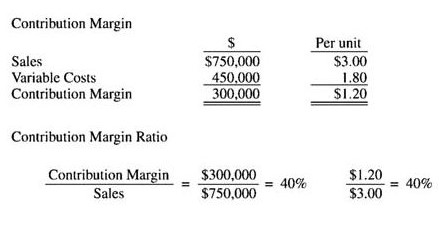## Break-even point

The break‐even point represents the level of sales where net income equals zero. In other words, the point where sales revenue equals total variable costs plus total fixed costs, and contribution margin equals fixed costs. Using the previous information and given that the company has fixed costs of \$300,000, the break‐even income statement shows zero net income.This income statement format is known as the contribution margin income statement and is used for internal reporting only.

The \$1.80 per unit or \$450,000 of variable costs represent all variable costs including costs classified as manufacturing costs, selling expenses, and administrative expenses. Similarly, the fixed costs represent total manufacturing, selling, and administrative fixed costs.

Break‐even point in dollars. The break‐even point in sales dollars of \$750,000 is calculated by dividing total fixed costs of \$300,000 by the contribution margin ratio of 40%.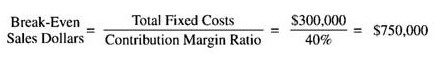Another way to calculate break‐even sales dollars is to use the mathematical equation.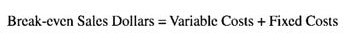In this equation, the variable costs are stated as a percent of sales. If a unit has a \$3.00 selling price and variable costs of \$1.80, variable costs as a percent of sales is 60% (\$1.80 ÷ \$3.00). Using fixed costs of \$300,000, the break‐even equation is shown below.The last calculation using the mathematical equation is the same as the break‐even sales formula using the fixed costs and the contribution margin ratio previously discussed in this chapter.

Break‐even point in units. The break‐even point in units of 250,000 is calculated by dividing fixed costs of \$300,000 by contribution margin per unit of \$1.20.The break‐even point in units may also be calculated using the mathematical equation where “X” equals break‐even units.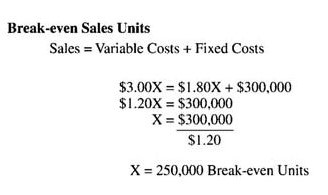Again it should be noted that the last portion of the calculation using the mathematical equation is the same as the first calculation of break‐even units that used the contribution margin per unit. Once the break‐even point in units has been calculated, the break‐even point in sales dollars may be calculated by multiplying the number of break‐even units by the selling price per unit. This also works in reverse. If the break‐even point in sales dollars is known, it can be divided by the selling price per unit to determine the break‐even point in units.## Targeted income

CVP analysis is also used when a company is trying to determine what level of sales is necessary to reach a specific level of income, also called targeted income. To calculate the required sales level, the targeted income is added to fixed costs, and the total is divided by the contribution margin ratio to determine required sales dollars, or the total is divided by contribution margin per unit to determine the required sales level in units.Using the data from the previous example, what level of sales would be required if the company wanted \$60,000 of income? The \$60,000 of income required is called the targeted income. The required sales level is \$900,000 and the required number of units is 300,000. Why is the answer \$900,000 instead of \$810,000 (\$750,000 [break‐even sales] plus \$60,000)? Remember that there are additional variable costs incurred every time an additional unit is sold, and these costs reduce the extra revenues when calculating income.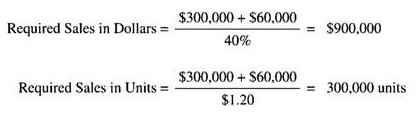This calculation of targeted income assumes it is being calculated for a division as it ignores income taxes. If a targeted net income (income after taxes) is being calculated, then income taxes would also be added to fixed costs along with targeted net income.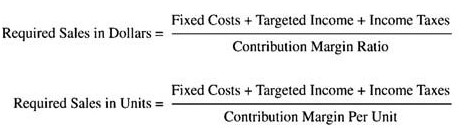Assuming the company has a 40% income tax rate, its break‐even point in sales is \$1,000,000 and break‐even point in units is 333,333. The amount of income taxes used in the calculation is \$40,000 ([\$60,000 net income ÷ (1 – .40 tax rate)] – \$60,000).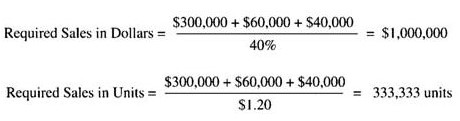A summarized contribution margin income statement can be used to prove these calculations.

## One thought on “Cost Volume Profit Analysis”

Please send Question paper of AKTU 4th SEM paper to intactquiz@gmail.com

X
error: Content is protected !!